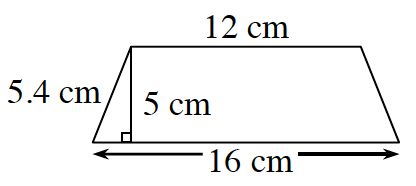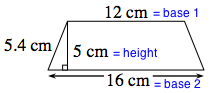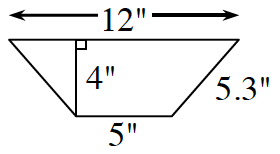### Home > CC1MN > Chapter 6 Unit 6 > Lesson CC1: 6.1.1 > Problem6-8

6-8.

Find the area of each of these trapezoids. Show all of your steps.

1.The equation for the area of a trapezoid is:

$\text{Area}=\frac{1}{2}(\text{base } 1 + \text{base }2)(\text{height})$

Locate the different parts of the equation on the trapezoid.
Then substitute the values and solve the equation to calculate the area.$\text{Area}=\frac{1}{2}(12+16)(5)$

$\text{Area} = 70\text{ square cm}$

1.See part (a).